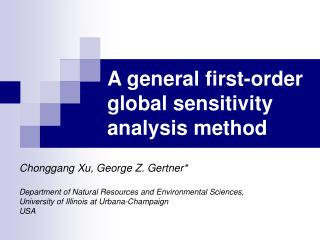DownloadDownload PresentationA general first-order global sensitivity analysis method

# A general first-order global sensitivity analysis method

Download Presentation## A general first-order global sensitivity analysis method

- - - - - - - - - - - - - - - - - - - - - - - - - - - E N D - - - - - - - - - - - - - - - - - - - - - - - - - - -
##### Presentation Transcript

1. A general first-order global sensitivity analysis method Chonggang Xu, George Z. Gertner* Department of Natural Resources and Environmental Sciences, University of Illinois at Urbana-Champaign USA

2. Uncertainty & Sensitivity analysis Techniques • Design of experiments method • Sampling-based method • Fourier Amplitude Sensitivity Test (FAST) • Sobol’s method • ANOVA method • Moment independent approaches

3. FAST Search function: Fourier transformation: Sum spectrum Variance decomposition:

4. Computationally efficient global sensitivity analysis method; Suitable for nonlinear and non-monotonic models; Aliasing effects for small sample sizes(frequency interference) ; Suitability for only models with independent parameters; FAST advantages & limitations

5. Real applications • Dependence among parameters; • Complex model with many parameters, which needs much computation times and large sample sizes in traditional FAST;

6. FAST improvement • Reorder  independent parameters limitation; • Random Balance Design (Tarantola et al. 2006)  commom frequency for all parameters and permuting  Overcome aliasing effect limitation; ↑ for each parameter. Tarantola, S, Gatelli, D, Mara, TA. Random balance designs for the estimation of first order global sensitivity indices. Reliability Engineering and System Safety, 2006; 91(6): 717-727.

7. Details of synthesized FAST • Xu, C. and G.Z. Gertner 2007. A general first-order global sensitivity analysis method. Reliability Engineering and System Safety (In press).

8. Reorder for correlation: Model Y = x1+ x2, correlation=0.7, characteristic frequency is 5 and 23 respectively. Independent sample order Correlated sample order of X1 Response Variable Y

9. Reorder for correlation: Model Y = x1+ x2,correlation=0.7,common characteristic frequency is 5 for both x1 and x2 Correlated sample order of X1 Independent sample order Response Variable Y

10. correlation=0.0 correlation=0.2 correlation=0.5 correlation=0.9

11. FAST sample with common characteristic frequency 6) Sample for FAST analysis of x1 Reordered sample 5) 3) Model outputs based on reordered sample 4) 2) 5) 1) Sample for the common variable s Sample for FAST analysis of x2 6)

12. Maximum Harmonic order selection Scaled characteristic spectrum 1. Random balance design may introduce random error. 2. Assume that the low characteristic amplitudes at high harmonic order are more susceptible to the random error than relatively high characteristic amplitudes at a low harmonic order .

13. Simulated annealing refinement for correlated samples PAR is photosynthetic active radiation

14. TEST CASES Synthesized FAST specification for test cases

15. Test case one: Y=2x1+3x2 ,where x1 and x2 are standard normally distributed with a Pearson correlation coefficient of 0.7 SFAST is synthesized FAST Circles are analytical

16. Test case two:(Lu and Mohanty, 2001) Rank correlation Circles are based on correlation ratio method by Saltelli(2001) based McKay’s one-way ANOVA. Nonparametric method suitable for nonlinear and monotonic models. 50,000 model runs (=100 replications x 500 samples)

17. Test case three: (G-function of Sobol’. Non-monotonic test model) Rank correlation Circles are based on correlation ratio method . 50,000 model runs (=100 replications x 500 samples)

18. Test case four: World 3 Model(Meadows et al., 1992)

19. Parameter uncertainty specification Rank correlation of .6 between x3 and x4 and.4 between x5 and x6

20. x3 x2 x5 x4 Sensitivity Year (Correlation ratio method (CRM). 50,000 model runs (=100 replications x 500 samples)

21. Assume correlation Assume Independence

22. ANPP CO2 Landscape composition Temperature LANDIS-II PnET-II SEP Precipitation Application: Uncertainty in forest landscape response to global warming PnET-II is a forest ecosystem process model (LINKAGES) is a forest GAP model LANDIS is a spatially dynamic forest landscape model)

23. (a) (b) (c) (d) Example of data after 1994 is based on prediction by Canadian Climate Center (CCC) in the Phase-II Vegetation-Ecosystem Modeling and Analysis Project (VEMAP). Data before 1994 is historical data. We assume the climate stabilizes after year 2099.

24. Parameter uncertainty PAR is photosynthetic active radiation

25. Rank correlation structure PAR is photosynthetic active radiation

26. Uncertainty Aspen-birch Maple-ash Uncertainty Pine Spruce-fir Simulation year

27. Sensitivity Aspen-birch Maple-ash Sensitivity Pine Spruce-fir Simulation year

28. Conclusion • Proposes a general first-order global sensitivity approach for linear/nonlinear models with as many correlated or uncorrelated parameters as the user specifies; • FAST is computationally efficient and would be a good choice for uncertainty and sensitivity analysis for models with correlated parameters;

29. Conclusion • Proposes a general first-order global sensitivity approach for linear/nonlinear models with as many correlated or uncorrelated parameters as the user specifies; • FAST is computationally efficient and would be a good choice for uncertainty and sensitivity analysis for models with correlated parameters;

30. Thank You!